4402.0 - Childhood Education and Care, Australia, June 2011
ARCHIVED ISSUE Released at 11:30 AM (CANBERRA TIME) 04/05/2012
 Page tools: .mffeedback,#pjs { display :none; } Enable Javascript to Print PagesPrint AllEmail Notification Explanatory Notes Glossary Abbreviations Data Comparison (Appendix) Quality Declaration Data quality (Technical Note) Data Source TECHNICAL NOTE DATA QUALITY ESTIMATION PROCEDURE 1 Estimates of children are derived using a ratio estimation procedure which ensures that estimates conform to an independently estimated state by age by sex distribution of children in the population, rather than to the state by age by sex distribution within the sample itself. Estimates of families conform to an independently estimated state by household composition distribution in the population, where household composition was defined by the number of adults and children within a household. RELIABILITY OF ESTIMATES 2 Since the estimates in this publication are based on information obtained from a sample, they are subject to sampling variability. That is, they may differ from those estimates that would have been produced if all dwellings had been included in the survey. One measure of the likely difference is given by the standard error (SE), which indicates the extent to which an estimate might have varied by chance because only a sample of dwellings (or households) was included. There are about two chances in three (67%) that a sample estimate will differ by less than one SE from the number that would have been obtained if all dwellings had been included, and about 19 chances in 20 (95%) that the difference will be less than two SEs. 3 Another measure of the likely difference is the relative standard error (RSE), which is obtained by expressing the SE as a percentage of the estimate. The RSE is a useful measure in that it provides an immediate indication of the percentage errors likely to have occurred due to sampling, and thus avoids the need to refer also to the size of the estimate: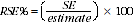4 RSEs for the 2011 Childhood Education and Care Survey have been calculated using the Jackknife method of variance estimation. This involves the calculation of 60 'replicate' estimates based on 60 different subsamples of the obtained sample. The variability of estimates obtained from these subsamples is used to estimate the sample variability surrounding the estimate. 5 RSEs of all the estimates in this publication are included in the Data Cubes released as part of the publication and available from the Downloads tab. 6 In this publication, only estimates (numbers and proportions, means and medians) with RSEs less than 25% are considered sufficiently reliable for most purposes. Estimates with larger RSEs have been included and are preceded by an asterisk (e.g. *13.5) to indicate they are subject to high sample variability and should be used with caution. Estimates with RSEs greater than 50% are preceded by a double asterisk (e.g.**2.1) to indicate that they are considered too unreliable for general use. CALCULATION OF STANDARD ERROR 7 SEs can be calculated using the estimates (counts or proportions) and the corresponding RSEs. For example, in Table 1 the estimated number of children aged 0-12 years who had usual child care arrangements was 1,902,700 and the associated RSE is 1.7%. The SE is calculated by: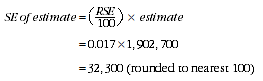8 Therefore, there are about two chances in three that the actual number of children aged 0-12 with usual child care arrangements was in the range of 1,870,400 to 1,935,000 and about 19 chances in 20 that the value was in the range 1,838,100 to 1,967,300. This example is illustrated in the diagram below.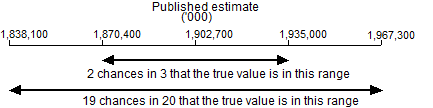PROPORTION AND PERCENTAGES 9 Proportions and percentages formed from the ratio of two estimates are also subject to sampling errors. The size of the error depends on the accuracy of both the numerator and the denominator. A formula to approximate the RSE of a proportion is given below. The formula is only valid when the numerator is a subset of the denominator: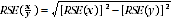10 As an example, using estimates from Table 1, of the 496,000 children aged 0-12 years who usually attended long day care, 6.6% (19,100) were aged under 1. The RSE for 496,000 is 3.1% and the RSE for 19,100 is 13.8%. Applying the above formula, the RSE for the proportion of children under the age of 1 who attended long day care is: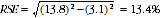11 Therefore, the SE for the proportion of children aged 0-12 years who usually attended long day care is 0.9 percentage points (=(13.4/100) x 6.6). Hence, there are about two chances in three that the proportion of children aged 0-12 years who usually attended long day care is between 5.7% and 7.5%, and 19 chances in 20 that the proportion is between 4.8% and 8.4%. DIFFERENCES 12 Published estimates may also be used to calculate the difference between two survey estimates (of numbers or proportions). Such an estimate is subject to sampling error. The sampling error of the difference between two estimates depends on their SEs and the relationship (correlation) between them. An approximate SE of the difference between two estimates (x-y) may be calculated by the following formula: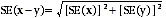13 While the above formula will be exact only for differences between separate and uncorrelated (unrelated) characteristics of subpopulations, it is expected that it will provide a reasonable approximation for all differences likely to be of interest in this publication. SIGNIFICANCE TESTING 14 A statistical significance test for any of the comparisons between estimates over time was performed to determine whether it is likely that there is a difference between the corresponding population characteristics. The standard error of the difference between two corresponding estimates (x and y) can be calculated using the formula above. This standard error is then used to calculate the following test statistic: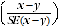15 If the value of this test statistic is greater than 1.96 then we may say there is good evidence of a real difference in the two populations with respect to that characteristic. Otherwise, it cannot be stated with confidence that there is a real difference between the populations. 16 The imprecision due to sampling variability, which is measured by the SE, should not be confused with inaccuracies that may occur because of imperfections in reporting by respondents and recording by interviewers, and errors made in coding and processing data. Inaccuracies of this kind are referred to as non-sampling error, and they occur in any enumeration, whether it be a full count or sample. Every effort is made to reduce non-sampling error to a minimum by careful design of questionnaires, intensive training and supervision of interviewers, and efficient operating procedures. Document Selection These documents will be presented in a new window.